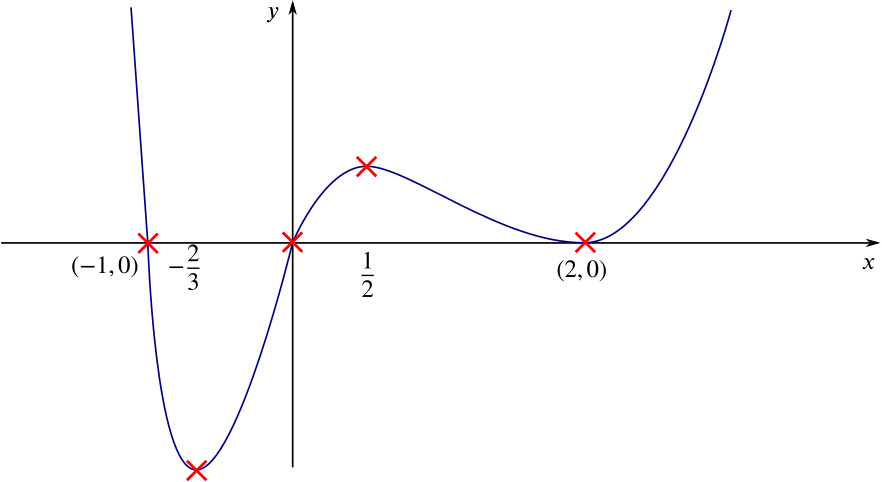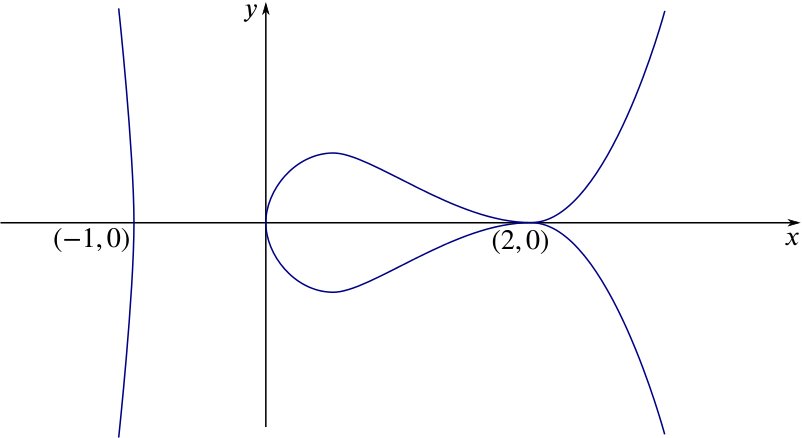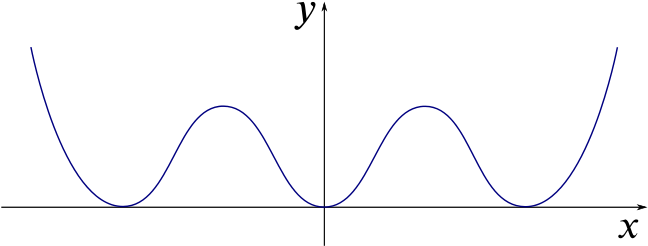Review question

# Can we sketch the graph of $y=x(x+1)(x-2)^4$? Add to your resource collection Remove from your resource collection Add notes to this resource View your notes for this resource

Ref: R8387

## Solution

The curve $C$ has equation $y=x(x+1)(x-2)^4.$ Show that the gradient of $C$ is $(x-2)^3(6x^2+x-2)$ and find the coordinates of all the stationary points.

We can write $y=(x^2+x)(x-2)^4$ before differentiating using the product and chain rules. This yields \begin{align*} \frac{dy}{dx}&=(2x+1)(x-2)^4+4(x^2+x)(x-2)^3 \\ &=(x-2)^3\left[(2x+1)(x-2)+4x(x+1)\right] \\ &=(x-2)^3(6x^2+x-2). \end{align*}
Alternatively, we could use the Product Rule for three functions, that is, if $y = uvw$, then $y' = u'vw + uv'w + uvw'$. \begin{align*} \frac{dy}{dx}&=(x+1)(x-2)^4+x(x-2)^4+4x(x+1)(x-2)^3 \\ &=(x-2)^3\left[(x+1)(x-2)+x(x-2)+4x(x+1)\right] \\ &=(x-2)^3\left[x^2-x-2+x^2-2x+4x^2+4x\right] \\ &=(x-2)^3(6x^2+x-2). \end{align*}

To find the $x$ coordinates of the stationary points we solve $\frac{dy}{dx}=(x-2)^3(6x^2+x-2)=0 = (x-2)^3 (3x+2)(2x-1),$ which has solutions given by $x=2 \text{ or } x=-\frac{2}{3} \text{ or } x=\frac{1}{2}.$ Substituting these values back in to $y$ we find the stationary points of $C$ are at $(2,0), \left(\frac{1}{2}, \frac{243}{64}\approx 3.8\right), \left(-\frac{2}{3}, -\frac{8192}{729}\approx-11.2\right).$

Determine the nature of each stationary point and sketch $C$.

As this function is a degree six polynomial (sextic) with positive coefficient of $x^6$ we know that for $x$ large and negative it is decreasing, and for $x$ large and positive it is increasing. Between these values the curve undulates so the first and last stationary points must be minima and the middle one a maximum.

Alternatively we could calculate the gradient at the integer values $x= -1, \: 0, \: 1, \:$ and $\: 3$ to verify the nature of the points.

We also know where all the $x$ intercepts are, and have calculated the stationary points: joining the dots gives us a sketch of our curve.A sketch of the graph of $y=x(x+1)(x-2)^4$

To determine the nature of the stationary point, we might be tempted to differentiate again and find the sign of this at the points where $\dfrac{dy}{dx} = 0$: $\frac{d^2y}{dx^2}=3(x-2)^2(6x^2+x-2)+(x-2)^3(12x+1).$

If we choose to do this we should notice that if $\dfrac{dy}{dx} = 0$, then the first term here is bound to be zero, which reduces the workload, but is still more effort than necessary.

We might also think that at $x=2$ there is a point of inflection because $\frac{d^2y}{dx^2}=0$ here. We can see from the expression for $y$ that the curve has a quadruple root at $x=2$, so we expect the curve to touch the $x$ axis here. (Note that a triple root would indicate a point of inflection.)

In separate diagrams draw sketches of the curves whose equations are:

1. $y^2=x(x+1)(x-2)^4$;

2. $y=x^2(x^2+1)(x^2-2)^4$.

In each case, you should pay particular attention to the points where the curve meets the $x$ axis.

To sketch the graph of (i), first notice that we must have $x(x+1)(x-2)^4 \geq 0$ in order for $y$ to take real values. This means that (i) is not defined for the region $-1 < x <0$.

The $x$-intercepts are the same, and the general shape will be similar to our first graph.

The difference is that every original $y$-value needs to be square-rooted for this graph, which will therefore be symmetrical about the $x$ axis. This leads us toA sketch of the graph of $y^2=x(x+1)(x-2)^4$

For sketch (ii), first notice that the roots for $y = 0$ have changed, that is, the $x$-intercepts will be different.

Also, notice that $y\geq 0$ for all $x$, and that we will have symmetry about the $y$ axis, since swapping $-x$ for $x$ gives the same $y$-value. .

We see that the roots are at $x=0$ and $x=\pm\sqrt{2}$, and now we have all we need to sketch the graph.A sketch of the graph of $y=x^2(x^2+1)(x^2-2)^4$